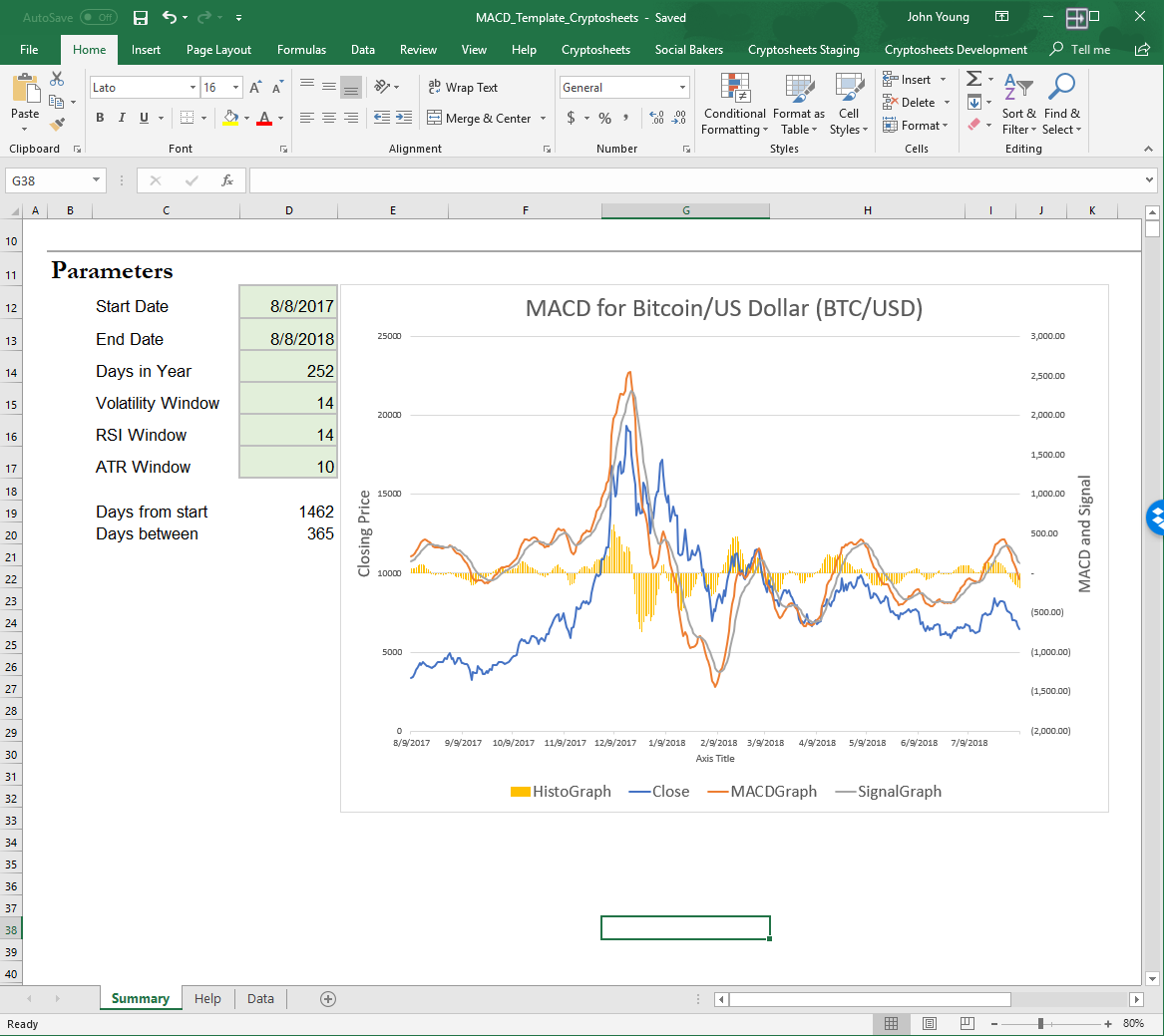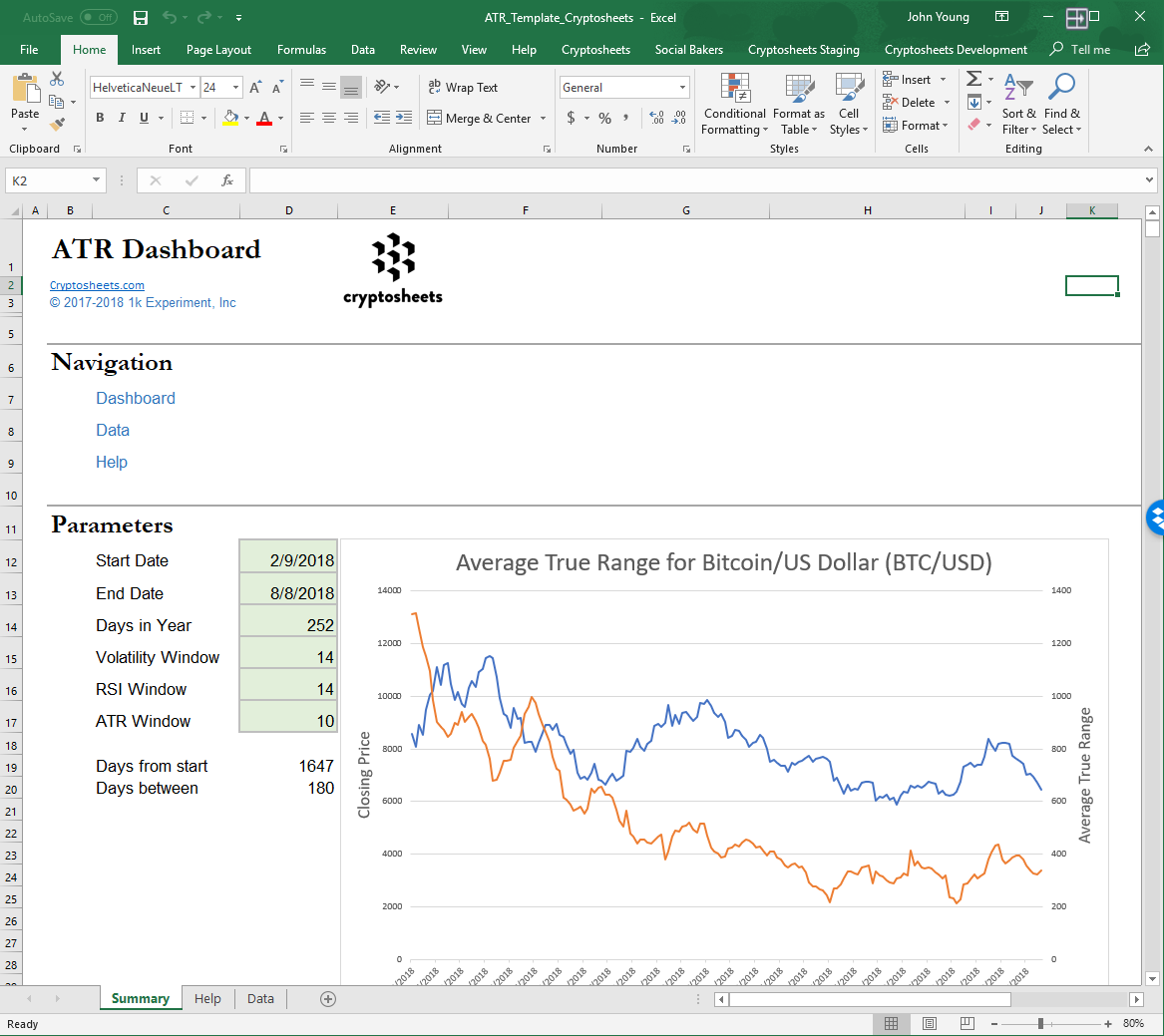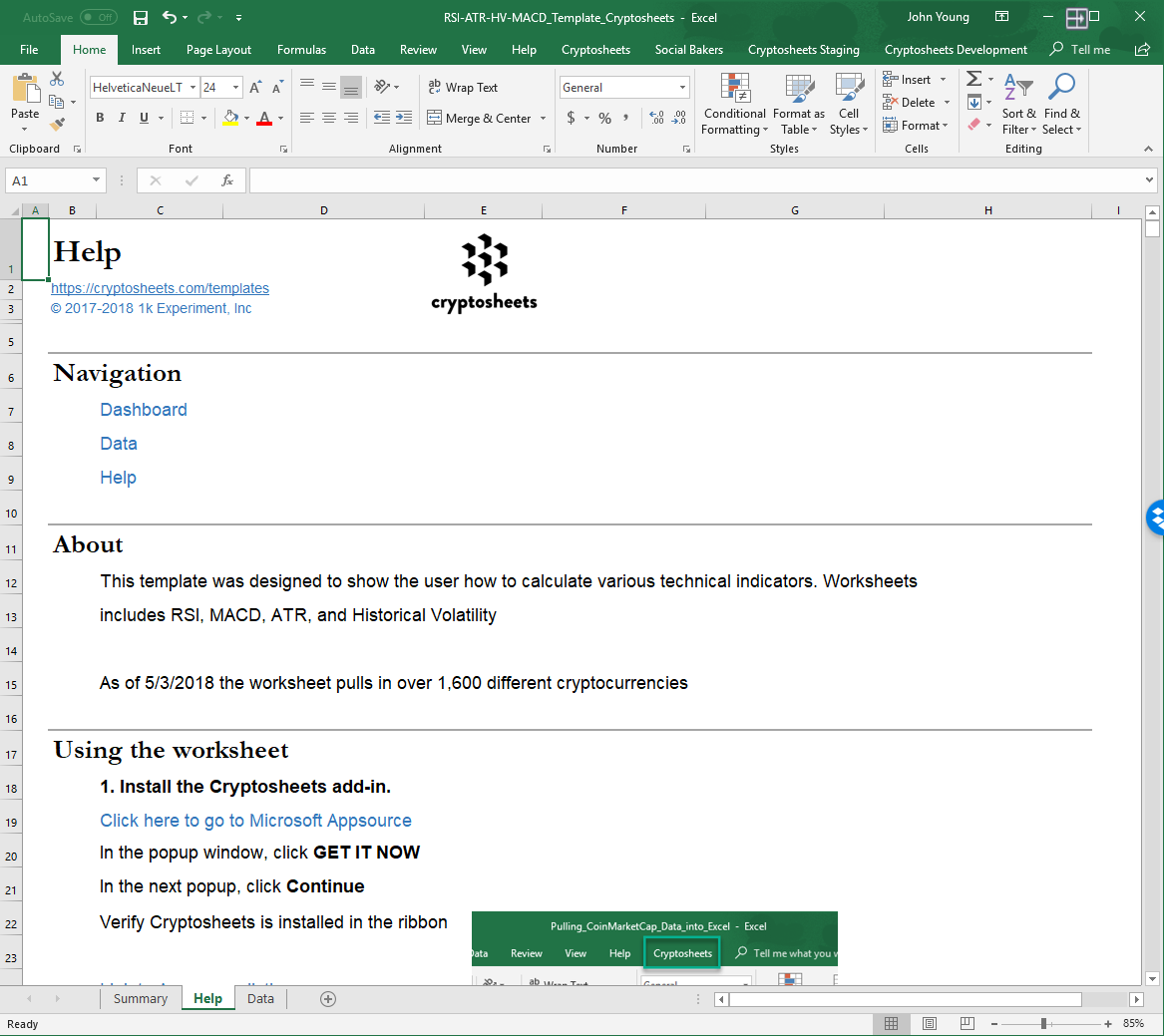# MACD Template

Moving average convergence divergence (MACD) is a trend-following momentum indicator that shows the relationship between two moving averages of prices. The MACD is calculated by subtracting the 26-day exponential moving average (EMA) from the 12-day EMA.

The template utilizes Cryptocompare 5-year OHLCV data for the calculations.•••